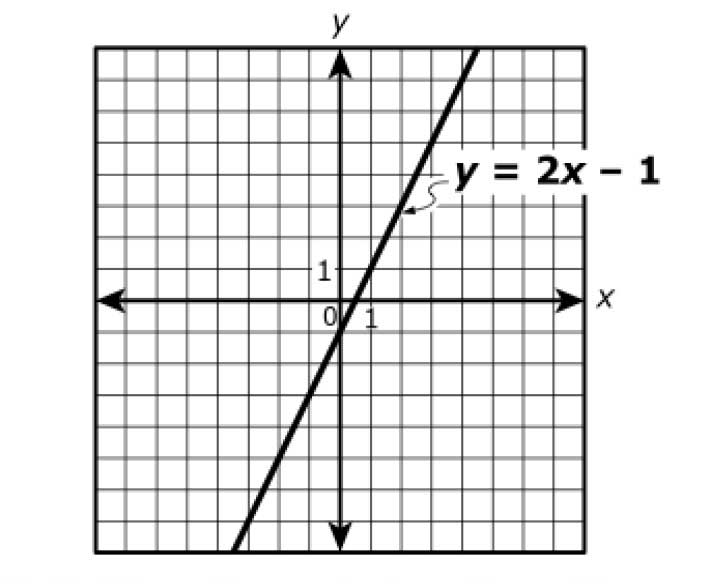Monday, October 18, 2021# Algebra 1 PARCC: points on a line

-

#### The following multiple-select question, explained here in hopes of helping algebra 1 students and their parents in Maryland and Illinois prepare for the PARCC test near the end of this school year, appears on the released version of PARCC’s Spring 2016 test for algebra 1 (#3):The graph of the function y = 2x – 1 is shown in the coordinate plane.Which lists contain only points that lie on the graph of y = 2x – 1 ?

A.   (0, –1), (15, 29), (2, 3)

B.   (2000, 1999), (3, 5)

C.   (–2, –3), (–5, –9), (0, 0)

D.   (–0.5, –2), (0.3, –0.4), (0.5, 0)

E.$(\frac{1}{4}\textrm{, }-\frac{1}{2})\textrm{, }(\frac{4}{5}\textrm{, }\frac{3}{5})$

Correct answer: A, D, and E should be selected.

Common Core Math Content High School, Algebra, Reasoning with Equations & Inequalities:

The question tests students’ understanding of Common Core standards in high school algebra, specifically those under reasoning with equations and inequalities, which require students to be able to (REI.D) “represent and solve equations and inequalities graphically” by (REI.D.10) understanding that “the graph of an equation in two variables is the set of all its solutions plotted in the coordinate plane, often forming a curve (which could be a line).”

In this problem, students are shown a line in the coordinate plane and given the equation for that line. The only reasoning required is the understanding that both the equation and the plotted line are different representations of the same function. If a point works in the equation or on the line, it’s then a correct answer to the question.

Solution strategy (there are others)

Plug each (x, y) point into the equation and see if it works.$y = 2x-1$$(-1) = 2(0)-1 \textrm{ ... yes.}$$(29) = 2(15)-1 \textrm{ ... yes.}$$(3) = 2(2)-1 \textrm{ ... yes.}$$(1999) = 2(2000)-1 \textrm{ ... no.}$$(5) = 2(3)-1 \textrm{ ... yes.}$$(-3) = 2(-2)-1 \textrm{ ... no.}$$(-9) = 2(-5)-1 \textrm{ ... no.}$$(0) = 2(0)-1 \textrm{ ... no.}$$(-2) = 2(-0.5)-1 \textrm{ ... yes.}$$(-0.4) = 2(0.3)-1 \textrm{ ... yes.}$$(0) = 2(0.5)-1 \textrm{ ... yes.}$$(-\frac{1}{2}) = 2(\frac{1}{4})-1 \textrm{ ... yes.}$$(\frac{3}{5}) = 2(\frac{4}{5})-1 \textrm{ ... yes.}$

## Analysis of this question and online accessibility

The question is valid in that it tests students’ ability to reach conclusions about points that lie on the graph of a linear function. Note that the function used on the test may not be a linear one, as it is in this sample problem. Students are required to be able to answer questions like this for other function types as well. The quickest way to do that is to plug in the values, once you find the function in the form y = f(x).

The sample problem here provided both a graph of the function (partial) and the function itself. It’s possible that students will be required to determine the equation given only a graph of the function. For linear functions, that can be done if the y-intercept and slope can be determined by plugging those values into the slope-intercept form of the equation, y = mx + b.

Multiple-select questions, as PARCC calls them, where more than one answer can be selected from several options, can be delivered online or on paper. Reliability, validity, and fairness performance should be equivalent, regardless of the delivery mode.

No special accommodation challenges can be identified with this question, so the question is considered fair.

## Resources for further study

Knowledge of the domain and range of functions, found in the high school algebra standards for functions, is implicit in the Common Core standard tested with this question, as students identify values in the domain and range of a linear function.

Purple Math, developed by Elizabeth Stapel, a math teacher from the St Louis area, has several pages about functions, their domains, and ranges, beginning here.

The Khan Academy, developed by Sal Khan, an engineer who has created a library of thousands of video lessons, has an old video, which still works perfectly well, that teaches students how to determine if a given point lies on the graph of a function. His series for this skill starts here, providing another possible solution strategy.

Ron Larson, Laurie Boswell, Timothy D Kanold, Lee Stiff. Algebra 2, Illinois edition. Evanston, Ill.: McDougal Littell, a division of Houghton Mifflin Company, 2008. The book is used in several algebra classes taught in Illinois high schools.

Foerster, Paul A. Algebra and Trigonometry: Functions and Applications, revised edition. Addison-Wesley, 1980, 1984. The book is used in several algebra classes taught in middle and high schools in both Illinois and Maryland.

## Purpose of this series

To help algebra 1 students and their parents prepare for the PARCC test in math, as administered in at least six states, or to just master content on that test, we provide an analysis of every algebra 1 math problem PARCC released in 2016. The series can be found here.Paul Katulahttps://news.schoolsdo.org
Paul Katula is the executive editor of the Voxitatis Research Foundation, which publishes this blog. For more information, see the About page.

### Now US News ranks elementary, middle schools

0
A magazine has launched national and state rankings for elementary and middle schools based on tests. Good idea or irrelevant?